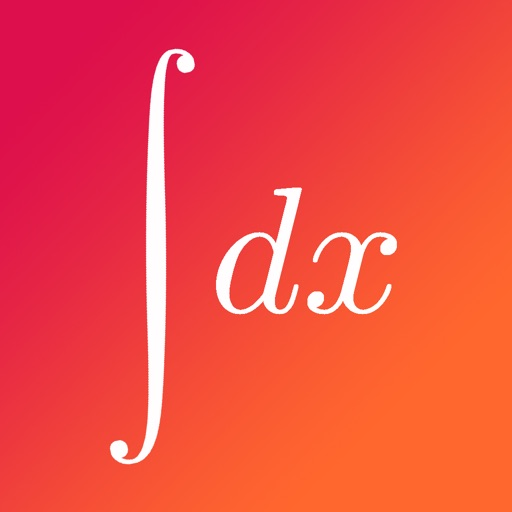## Calculus X is the most effective study tool for calculus at the AP or intro college level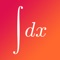# Calculus X: College and AP Calc Test Prep and Tools

by Knowvio LLC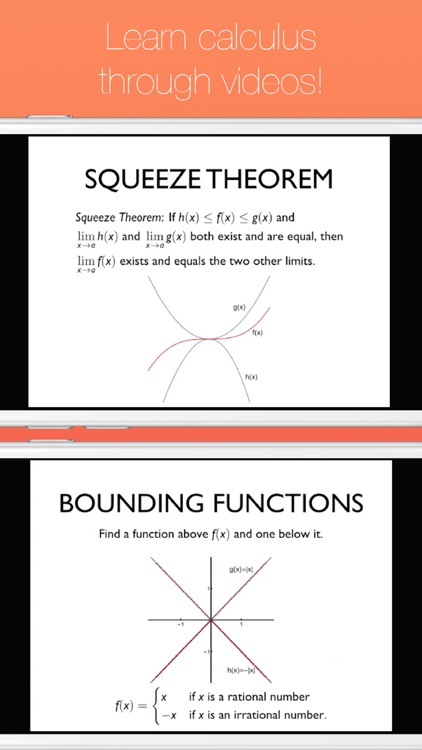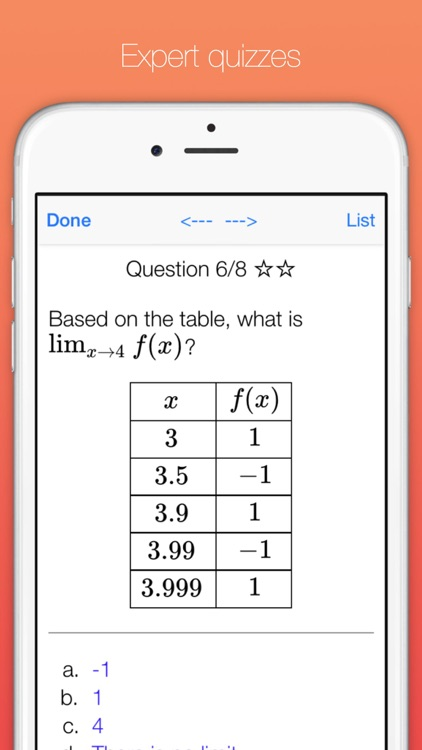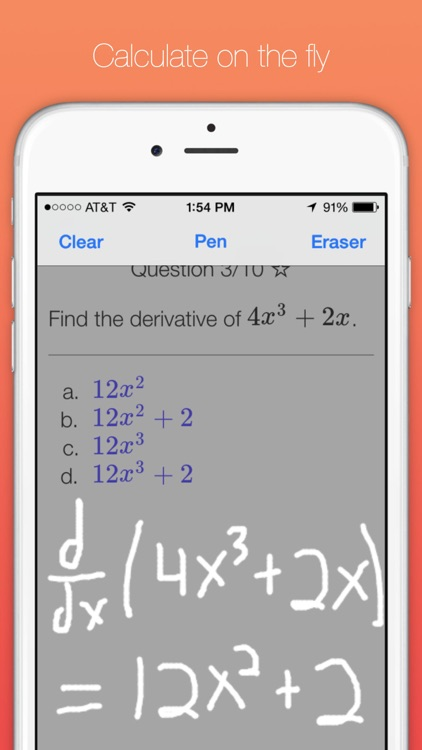Calculus X is the most effective study tool for calculus at the AP or intro college level. It integrates free response questions, concise videos, quizzes, formula sheets, scratch pad and references to make the study process efficient, easy and mobile.### App Details

Version
1.4.1
Rating
(24)
Size
30Mb
Genre
Education Puzzle
Last updated
August 16, 2016
Release date
September 17, 2015

• \$4.99

### App Screenshots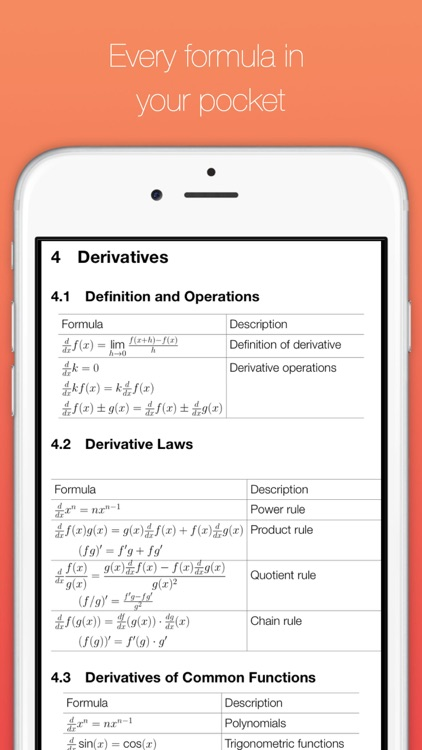### App Store Description

Calculus X is the most effective study tool for calculus at the AP or intro college level. It integrates free response questions, concise videos, quizzes, formula sheets, scratch pad and references to make the study process efficient, easy and mobile.

If you’re a busy student, finding enough time to study for each calculus exam is a challenge. We have used the latest developments in educational psychology to build an app that maximizes productivity and helps you learn and do calculus as quickly as possible.

WHAT’S INCLUDED:

-Over 800 multiple choice questions with detailed solutions.
These conceptual based questions help you to learn calculus in a deep and intuitive way. They can all be answered without a calculator, pen or paper; making the study process as mobile as possible.

-Over 30 beautiful 720p videos which solve free response style questions in step-by-step detail.
These concise videos were designed specifically for the mobile device and solve the problems clearly and systematically in order to develop your problem solving intuition. They use narration and detailed visual guidance to increase understanding of the solution method. More video content coming soon.

-Extensive formula sheets accompany each chapter for quick access to all formulas from intro calculus, and all the necessary formulas from pre-calculus. Plus referenc e

-Fun and easy-to-use scratch pad allows you to do calculations on the fly, without the need for pencil and paper.

-Reference materials include: trigonometric identities, differentiation rules, limit laws, key theorem statements, derivatives of all common functions, and more.

WHO THE APP IS FOR:

- Students taking Calculus 1 or Calculus 2 in college
- Students taking AP calculus in high school
- Students studying for exams in different fields who need to review calculus
- Anybody interested in learning calculus

TOPICS COVERED:

---Limits---

Limits Intuition
Limit Operations
Factoring and Limits
Rationalizing and Limits
The Squeeze Theorem
Vertical Asymptotes
Horizontal Asymptotes
Relative Magnitude
One-Sided Limits

---Continuity---

Continuity Intuition
Limit Definition of Continuity
Examples of Continuous Functions
The Composition Theorem
The Intermediate Value Theorem

---Derivatives---

Derivatives Intuition
Limit Definition of the Derivative
Derivative Operations
The Power Rule
The Quotient Rule
Differentiating Trigonometric Functions
The Chain Rule
Differentiating Exponential and Logarithmic Functions
Logarithmic Differentiation
Implicit Differentiation
Tangent Lines
Related Rates
Linear Approximation
Higher Order Derivatives
Maxima and Minima
Optimization
The Mean Value Theorem
L’Hopital’s Rule

---The Integral ---

Antiderivatives
Riemann Sums
Summation Notation
Definition of the Integral
Fundamental Theorem of Calculus
Properties of the Integral
Integral Versus Area
The Integral Function

--Integration Techniques---

Polynomial Integration
Exponential Integration
Trig function integration
Even and odd functions
U-substitution
Bounds and U-substitution
Multiple trig functions
Integration by parts
Trigonometric substitution
Partial fraction decomposition
Discontinuities and absolute values
Improper integrals

---Applications of Integration---

Arc Length
Differential Equations

---Series---

Convergence limit definition
Telescoping series
Geometric series
Harmonic series
p-series
Divergence test
Comparison test
Limit comparison test
Ration test
Root test
Integral test
Alternating series test
Absolute and conditional convergence

---Taylor Series---

Series with variables
Taylor series intuition
Partial Taylor series
Full Taylor series
Shifted Polynomials
Taylor series operations